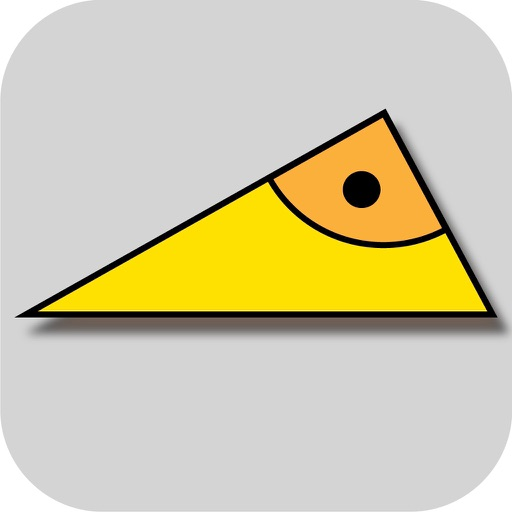## At school or for work, quickly and easily calculate the values of a right-angled triangle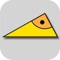# Right Triangle Solver

by Carsten Pohland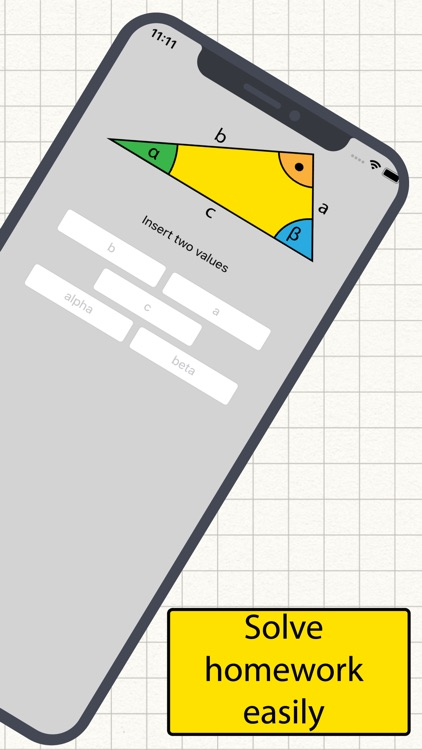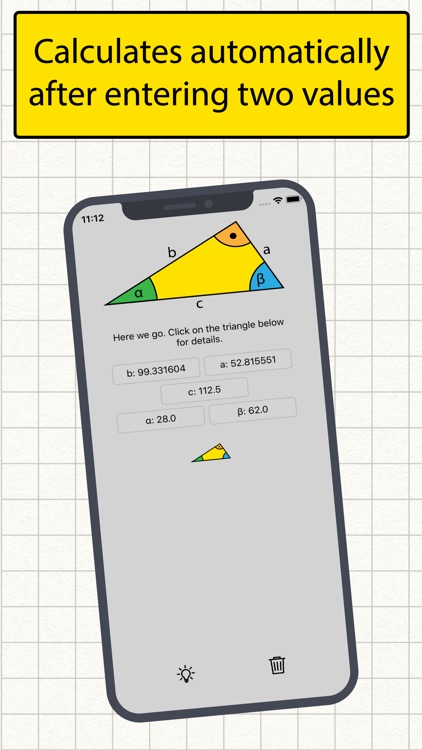At school or for work, quickly and easily calculate the values of a right-angled triangle? No problem!### App Details

Version
2.0.1
Rating
(6)
Size
12Mb
Genre
Education Utilities
Last updated
March 6, 2020
Release date
March 11, 2019

### App Screenshots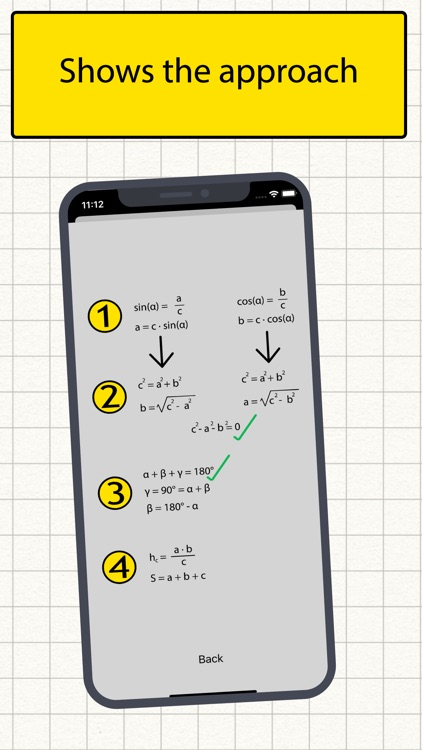### App Store Description

At school or for work, quickly and easily calculate the values of a right-angled triangle? No problem!

You don't remember whether you have to calculate with the Pythagorean theorem or with trigonometric functions?
The app takes the decision for you. Just enter hypotenuse, cathetus, opposite, adjacent or an angle. With two values, all other values can be calculated at the right-angled triangle. As soon as two values are entered, the app calculates automatically.

You want to study for school, class test or exam in math or geometry? The app shows a possible solution and the formulas. So homework is quickly solved,

Quickly perform calculations on a right-angled triangle while working and don't have a calculator with sine, cosine, tangent or root at hand? Never mind. The app solves the task for you reliably and quickly.

The app draws the right-angled triangle to scale and displays other values such as the height, circumference, area and angles in radians.

- For quick calculations at work or at school
- Understanding Pythagoras' theorem at last
- Applying trigonometric functions to a right-angled triangle
- Learning and understanding mathematics and geometry
- Draws the calculated triangle true to scale
- Also calculates the height, perimeter and area
- Shows the angles in arc dimension
- Using trigonometry and Pythagoras formulas
- Shows a possible solution of the calculation with the formulas
- Good aid for homework or for exam preparation
- Right-angled triangles for work quickly calculated
- Computer for trigonometry and Pythagoras
- The number of digits after the decimal point can be set

The solution path is displayed based on the entries with the formulas. An indispensable calculator app for homework and exam preparation. A trigonometry and Pythagoras calculator for work. An excellent tool thanks to its clear interface and intuitive operation.

Disclaimer:
AppAdvice does not own this application and only provides images and links contained in the iTunes Search API, to help our users find the best apps to download. If you are the developer of this app and would like your information removed, please send a request to [email protected] and your information will be removed.